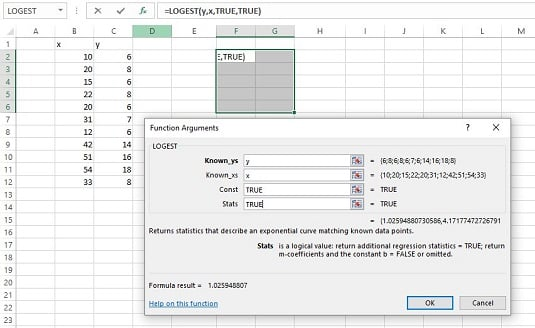##### Statistical Analysis with Excel For Dummies, 5th EditionIt's possible to have a relationship between two variables that's curvilinear rather than linear. The Excel function `LOGEST` estimates a and b for this curvilinear equation. The image below shows the `LOGEST` Function Arguments dialog box and the data for this example. It also shows an array for the results. Before using this function, you can attach the name x to B2:B12 and y to C2:C12.The Function Arguments dialog box for `LOGEST, along with the data and the selected array for the results.`

Here are the steps for this function:

1. With the data entered, select a five-row-by-two-column array of cells for `LOGEST`'s results. Select F4:G8.
2. From the Statistical Functions menu, select `LOGEST` to open the Function Arguments dialog box for `LOGEST`.
3. In the Function Arguments dialog box, type the appropriate values for the arguments. In the Known_y's box, type the cell range that holds the scores for the y-variable. For this example, that’s y (the name given to C2:C12). In the Known_x's box, type the cell range that holds the scores for the x-variable. For this example, it’s x (the name given to B2:B12). In the Const box, the choices are `TRUE` (or leave it blank) to calculate the value of a in the curvilinear equation or `FALSE` to set a to 1. Type `TRUE`. The dialog box uses b where you can use a. No set of symbols is standard. In the Stats box, the choices are `TRUE` to return the regression statistics in addition to a and b, `FALSE` (or leave it blank) to return just a and b. Type `TRUE`. Again, the dialog box uses b where you can use a and m-coefficient where b was used.
4. Important: Do not click OK. Because this is an array function, press Ctrl+Shift+Enter to put `LOGEST`’s answers into the selected array.
The image below shows `LOGEST`’s results. They’re not labeled in any way, so the labels are added for you in the worksheet. The left column gives you the exp(b) (more on that in a moment), standard error of b, R Square, F, and the SSregression. The right column provides a, standard error of a, standard error of estimate, degrees of freedom, and SSresidual.

About exp(b). `LOGEST`, unfortunately, doesn’t return the value of b – the exponent for the curvilinear equation. To find the exponent, you have to calculate the natural logarithm of what it does return. Applying Excel's LN worksheet function here gives 0.0256 as the value of the exponent.